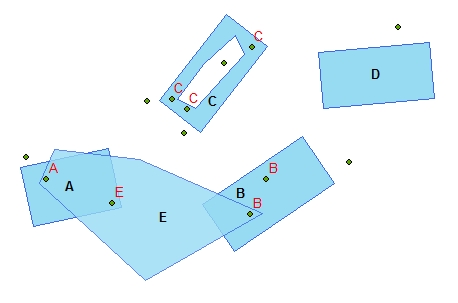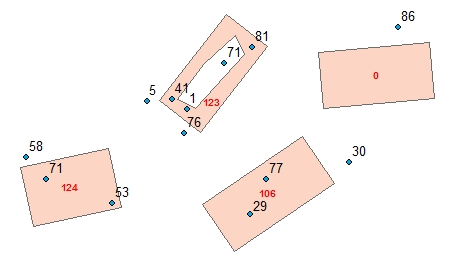## Spatial Join

Running programmatically

Joins the attributes of the Join Layer to the attribute table of the Target Layer based on spatial location.

### Inputs:

• Target Dataset - Point, Multipoint, Polyline or Polygon
• Join Dataset - Point, Multipoint, Polyline or Polygon
• Join Type
• Join Type = "One To Many" - one feature from the Join Dataset can be joined to many features of the target dataset, but each feature from the Target Dataset will receive the attributes of only ONE of the features from the Source Dataset:
• Point to Polygon - the polygon will get the attributes of the deepest point inside the polygon (farthest to the polygon boundary) or if there is no point inside the closest point to the polygon (within the search tolerance)

Example: Points to Polygons - Search Tolerance = 0• Point to Point, Point To Polyline, Point To Multipoint - the target will get the attributes of the closest polyline
• Polyline to Polygon - the polygon will get the attributes of the polyline with the longest intersection with the polygon or if there is no intersecting polyline the closest polyline to the polygon (within the search tolerance)

Example: Polylines to Polygons - Search Tolerance = 0• Polyline to Point, Polyline To Polyline, Polyline To Multipoint - the target will get the attributes of the closest point
• Polygon to Point - the point will get the attributes of the polygon:
• If the point is within the polygon - the polygon with largest distance to the boundary
• If the point is not in any polygon - the closest polygon within the search tolerance.

Example: Polygons to Points - Search Tolerance = 0 - Update Rule = "Sum"• Polygon to Polyline - the polyline will get the attributes of the polygon with longest intersection. If the polyline does not intersect any polygon - the closest polygon within the search tolerance.
• Polygon to Polygon - the polygon will get the attributes of the polygon with largest area of intersection. If the polygon does not intersect any source polygon - the closest polygon within the search tolerance.
• Join Type = "Many To Many" - the target feature will get the attributes of all source features within the search tolerance according to the user specified update rule for each field to be joined.

Example: Points to Polygons - Search Tolerance = 0• Cut-off distance (Search Tolerance) - the maximum distance for which the attributes of the features in the Join layer will be joined to the target features (in the units of the spatial reference of the Target Dataset)
• Join Fields - the fields from the Join Layer that will be added to the Target layer. If the Join Type is "One To Many" - the values of the joined feature will be stored. If the Join Type is "Many To Many" - the values for each joined field will be added according to the user defined update rules.
• Keep All Target Features - if selected all features of the target layer will be exported to the output dataset. If not selected only the target features that were joined to the source features will be saved in the output. This option is only valid to "One To Many" Join Type. If the Join Type is "Many To Many", all features of the target dataset are preserved.

Example: Points to Polygons - Search Tolerance = 0 Keep All Target Features = FALSE. The feature with no joins is missing in the output.• Add statistics fields - used only if the Join Type is "Many To Many". Statistcs for the Spatial Join will be added to the output attribute table depending on the type of the Target and Source datasets
• [ET_Count] - the number of source features joined to the target feature.
• [ET_CountIn] - if the target is polygon or polyline - the number of the joined features that intersect each target feature. Note that if the search tolerance is larger than 0 the number of the joined features might be larger than the number of intersecting features
• [ET_LengthIn] - if the target is polygon and the source polyline - the sum of the length of the polylines intersecting the target polygon
• [ET_LengthIn] - if the target and the source are polygons - the sum of the area of the polygons intersecting the target polygon

### Outputs:

• New layer
• The attributes of the target layer will be preserved in the output dataset
• The join fields from the Source layer will be added to the output and the values will be populated as described above
• If Add statistics fields = TRUE, statistics fields will be added to the output and the values will be populated as described above

(Go to TOP)

### Parameters

Expression Explanation
Function Name SpatialJoin
<Target Dataset> A String representing the target layer.
<Join Dataset> A String representing the join layer.
<output dataset> A String - the full name of the output layer.
< Join Type> A String representing the Join Type to be used. Valid values: "OneToMany" and "ManyToMany"
<CutOff Distance> A Double representing the Cut-Off Distance to be used. The units of the tolerance are the units of spatial reference of the Target Dataset
<Join Fields< A String representing a list (separator ";") of the fields to transfer together with the method for each field. Valid values - "Sum", "Max", "Min" for number fields and "First", "Last" for string fields. Example: "Field1 Sum; Field2 First; Field3 Min"
{Keep All} A Boolean indicated whether to keep all target features - see explanation above.
{Add Statistics} A Boolean indicated whether to add statistics fields - see explanation above.

### Running the function

ETGWPath used in the table below is the full path to ETGWRun.exe (E.G. "C:\Program Files\ETSpatial Techniques\ETGeo Wizards\ETGWRun.exe")

Language Syntax
Python subprocess.call([ETGWPath, "SpatialJoin", "Target Dataset", "Join Dataset", "output dataset", " Join Type", "CutOff Distance", "Join Fields", "Keep All", "Add Statistics"])
.NET using ETGWRun.exe StartInfo.FileName = ETGWPath
StartInfo.Arguments = "SpatialJoin" "Target Dataset" "Join Dataset" "output dataset" "Join Type" "CutOff Distance" "Join Fields" "Keep All" "Add Statistics"
.NET using ETGWOutX.dll SpatialJoin(Target Dataset,Join Dataset, output dataset, Join Type, CutOff Distance,Join Fields,Keep All,Add Statistics)
ArcPy arcpy.SpatialJoin(Target Dataset, Join Dataset, output dataset, "Join Type", "CutOff Distance", "Join Fields","Keep All", "Add Statistics")

Notes:

• <> - required parameter

• {} - optional parameter

• See examples for Python , .NET or ArcPy

• The argument separator for StartInfo.Arguments is space. If a string might contain a space, you need to double quote it.

(Go to TOP)